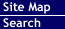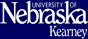Offered by Department of Mathematics and Statistics
College of Natural and Social Sciences

### MATH Courses

MATH 090 - Elementary Algebra - 3 hours
Prereq: none
Placement: ACT £ 16**
This course deals with elementary concepts of algebra which are usually taught at the 9th grade level. Emphasis is placed on developing functional competence in the several areas of algebra which are covered, and the content includes some practical applications. Not a General Studies course. Credit will not count toward any UNK degree.
MATH 101 - Intermediate Algebra - 3 hours
Prereq: MATH 090
Placement: 17 £ ACT £ 19** and 1 year of high school algebra
The course which includes a study of the properties of real numbers, polynomials, fundamental operations, factoring, exponents, and radicals, linear and quadratic equations, and other selected topics, all of which are necessary for the study of college algebra. Not a General Studies course.
MATH 102 - College Algebra - 3 hours
Prereq: MATH 101
Placement: ACT ³ 20** and 2 years of high school algebra
A college level algebra course which includes a study of linear equations and inequalities, relations and functions, graphing of linear and quadratic functions, polynomial and rational functions, logarithmic and exponential functions, systems of equations, matrices, sequences and series, and other selected topics all of which are necessary for the study of calculus.
MATH 102L - College Algebra Laboratory - 1 hour
Prereq: MATH 101
The lab provides the students with small group and cooperative learning experiences as well as the opportunity for additional problem and solutions experiences in a structural learning environment.
MATH 103 - Plane Trigonometry - 3 hours
Prereq: MATH 102
Placement: ACT ³ 22** and 2 years of high school algebra
Study of trigonometric functions.
MATH 104 - Concepts in Mathematics and Statistics - 3 hours
Prereq: MATH 101
Placement: ACT ³ 20** and 3 years of high school mathematics
An algebra course designed specifically for students going into elementary education. Many of the topics are similar to topics found in a traditional college algebra course. However, topics from the areas of probability and statistics have been included to give the prospective elementary teacher the necessary background to meet state and national curriculum standards for elementary mathematics.
MATH 106 - Mathematical Concepts - 3 hours
Prereq: MATH 101
Placement: ACT ³ 17** and 2 years of high school algebra
An enrichment course investigating the structure, aesthetics and philosophy of mathematics and its cultural relevance.
MATH 115 - Calculus I with Analytic Geometry - 5 hours
Prereq: MATH 102, MATH 103
Placement: ACT ³ 23** and 4 years of high school mathematics including 2 years of algebra, 1 year of geometry, and a senior level pre-calculus course.
Limits and continuity, differentiation of algebraic and trigonometric functions, elementary integration (with applications) of algebraic and trigonometric functions.
MATH 120 - Finite Mathematics - 3 hours
Prereq: MATH 102
Placement: ACT ³ 22 and 2 years of high school algebra
An introduction to modern mathematical concepts, with applications. Includes logic, set theory, probability, vectors, matrices, linear programming, and game theory.
MATH 123 - Applied Calculus I - 3 hours
Prereq: MATH 102
Placement: ACT ³ 22** and 4 years of high school mathematics including 2 years of algebra, 1 year of geometry, and a senior level pre-calculus course.
The concepts of calculus with emphasis on applications to the areas of business, biology, economics, and the social and behavioral sciences. Credit cannot be received for both MATH 115 and 123.
MATH 202 - Calculus II with Analytic Geometry - 5 hours
Prereq: MATH 115*
Placement: ACT ³ 25** and 1 year of high school calculus
A continuation of Math 115 including the differentiation and integration of transcendental functions, methods of formal integration with applications, series.
MATH 230 - Math for Elementary Teachers I - 3 hours
Prereq: MATH 104 or MATH 102 or permission
Placement: ACT ³ 20** and 4 years of high school mathematics including 2 years of algebra, 1 year of geometry, and a senior level math course.
Numeration, sets, relations, bases, and a detailed development of the number system from an elementary school point of view.
MATH 250 - Foundations of Math - 3 hours
Prereq: MATH 115* or MATH 123*
Topics of sets and symbolic logic are studied with the objective of using them in the detailed study of the nature of different types of proofs used in mathematics. Also, the processes of problem solving are studied for developing strategies of problem solving.
MATH 260 - Calculus III - 5 hours
Prereq: MATH 202* or equivalent preparation
A continuation of 202. Vector calculus, partial derivatives and multiple integrals.
MATH 305 - Differential Equations - 3 hours
Prereq: MATH 260*
Methods of solution and applications of common types of differential equations.
MATH 310 - College Geometry - 3 hours
Prereq: MATH 250*
Mathematical systems and re-examination of Euclidean geometry from an advanced viewpoint.
MATH 330 - Math for Elementary Teachers II - 3 hours
Prereq: MATH 230
Algebraic and geometric topics as related to elementary mathematics.
MATH 350 - Abstract Algebra - 3 hours
Prereq: MATH 250* or permission
An introduction to modern algebra, including a brief study of groups, rings, integral domains and fields.
MATH 399 - Internship - 1-4 hours
Prereq: none
On the job experience designed to complement the major. Internship experiences are available only in selected areas. Consult with the departmental advisor. MATH 399 is a credit/no credit course.
MATH 400/800P - History of Mathematics - 3 hours
Prereq: MATH 115*
An introduction to the history of mathematics from its primitive origins to modern-day mathematics.
MATH 404/804P - Theory of Numbers - 3 hours
Prereq: MATH 250* or permission
Properties of integers, congruencies, primitive roots, arithmetic functions, quadratic residues, and the sum of squares.
MATH 413/813P - Discrete Mathematics - 3 hours
Prereq: MATH 250*
A study of the evolution of the number systems from the naturals through the complex with emphasis on the complex. A detail study of the topics of discrete math including counting techniques, probability, graphs, trees and relations.
MATH 414/814P - Theory of Compound Interest - 3 hours
Prereq: MATH 115* or MATH 123*
A study of the forces of interest, accumulated values, present value factors, annuities certain, amortization schedules, sinking funds, bonds, and securities.
MATH 420/820P - Numerical Analysis - 3 hours
Prereq: MATH 260* or permission of instructor
The solution of nonlinear equations, interpolation and approximation, numerical integration, matrices and system of linear equations, and numerical solution of differential equations.
MATH 430/830P - Middle School Mathematics - 3 hours
Prereq: MATH 104* or permission
A study of additional topics found in grades 5-9 mathematics not covered in MATH 230, 330, e.g. algebra, probability, calculators, computer and statistics.
MATH 440/840P - Linear Algebra - 3 hours
Prereq: MATH 115*
Vector spaces, linear transformations, matrices, and determinants.
MATH 460/860P - Advanced Calculus I - 3 hours
Prereq: MATH 250*, MATH 260*
Functions, sequences, limits, continuity, differentiation and integration.
MATH 470/870P - Teaching of Secondary Mathematics - 3 hours
Prereq: MATH 260* or permission of instructor, admission to Teacher Education
Recent trends in the content of the mathematics curriculum and of procedures for the improvement of teaching mathematics in the high school.
MATH 490/890P - Special Topics in Mathematics - 1-3 hours
Prereq: none
Topics chosen from the areas of mathematics appropriate to the student's program and will involve both formal lectures and independent study.
MATH 495/895P - Independent Study in Mathematics - 1-3 hours
Prereq: MATH 260* or permission
An individual investigation by the student of topics not included in the normal mathematics offerings.
MATH 496/896P - Mathematics Seminar - 1 hour
Prereq: MATH 260* or permission
Topics not included in the normal mathematics offerings are presented by the students.

17 Feb 2005